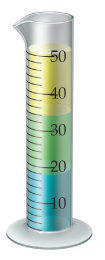# Problem: The following cylinder contains three liquids that do not mix with one another:water (density=1.0g/mL),vegetable oil (density=0.93g/mL),and mercury (density=13.5g/mL).Which liquid is which?

###### FREE Expert Solution
91% (140 ratings)
###### Problem Details

The following cylinder contains three liquids that do not mix with one another:
water (density=1.0g/mL),
vegetable oil (density=0.93g/mL),
and mercury (density=13.5g/mL).Which liquid is which?